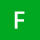Home
IT Knowledge
Inspiration
Languages
EN

# Java - random int between MIN MAX value - both inclusive

2 pointsFlimzy
356

How to generate random number in java between min and max value?
But I would like to create method to get number between min and max - both inclusive.
I don't like when I need to think if min or max is inclusive or exclusive :)
Let's say random number between 0 and 3:
[0, 3] - both inclusive (where 0 is min and 3 is max).

1 pointsFlimzy
356

Here we have Util class with small example if this method works as asked in questions. Both inclusive between 0 and 3.

[0, 3]

Code and output:

``````import java.util.Random;

public class RandomUtil {

private static final Random RANDOM = new Random();

public static int nextIntBetweenMinInclusiveMaxInclusive(int min, int max) {
return RANDOM.nextInt((max - min) + 1) + min;
}

public static void main(String[] args) {

for (int i = 0; i < 15; i++) {
int randomNumber = nextIntBetweenMinInclusiveMaxInclusive(0, 3);
System.out.println(randomNumber);
}
}
}``````

Output:

``````1
1
2
0
1
3
2
1
3
1
0
1
0
1
3``````

Like we can see, the method works, we get numbers between [0, 3] both inclusive.

Here is wiki article solution for this problem:

Java random number between 1 and 10 - both inclusive